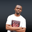Related Tags

euphoria
communitycreator

# How to calculate the similarity between two Euphoria sequenceNDUKWE CHIDERA K.

## Overview

The similarity between two sequence variables in Euphoria can be based on how close to being the same the items of such variables are.

The sim_index() method will calculate the similarity between the provided sequences.

### What is the sim_index() method?

The sim_index() method is part of the sequence.e module from the standard Euphoria library. It is used to calculate the similarity between two sequence variables. The output of the sim_index() can range between 0 and 1, where 0 and 1 are not inclusive in the range.

• The closer to 0 the output is, the more alike the two sequences are. Whereas the output would be closer to 1 the more different they are.

### Syntax

sim_index(A, B)



### Parameters

This function, as indicated in the syntax, accepts two parameters: A and B, whose similarity will be calculated.

### Returns

The more the two sequences are alike, the sim_index() method returns an atom closer to 0.

### The operational pattern of this method

The output gotten is weighted so that elements mismatched from the start are given a larger value/sim_index score. This implies that sequences closer to the beginning will be considered more unalike than those that differ towards their ends.

Note: If the values of two items are the same, the output will be 0, while a non-zero will indicate that they are not identical, and a larger value will show a larger difference.

### Example

We will calculate the sim_index score between a few sequence variables in the code snippet below.

include std/sequence.e

sequence seq_A, seq_B, seq_C, seq_D, seq_E, seq_F
atom output1, output2, output3

seq_A = "Deterrent"
seq_B = "Determine"
seq_C = {1,2,3,4}
seq_D = {1,2,3,4}
seq_E = "PESSIMISM"
seq_F = "OPTIMISM"

output1 = sim_index(seq_A,seq_B)
output2 = sim_index(seq_C, seq_D) -->output for this should be 0.0000
output3 = sim_index(seq_E,seq_F)

printf(1,"The similarity index between seq_A and seq_B is : %f",output1)
printf(1,"\nThe similarity index between seq_C and seq_D is : %f",output2)
printf(1,"\nThe similarity index between seq_E and seq_F is : %f",output3)


### Explanation

From the above snippet, we can see that the operation on line 12 has a value of 0 because they are similar and have the same value. In also comparing the outputs from the operations on lines 13 and 15, we can see how close the output is to 0 on line 13, unlike the one on line 15. This is because Deterrent and Determine are similar from the beginning whereas PESSIMISM and OPTIMISM are different.

• Line 1: We include the sequence.e.

• Lines 3 and 4: We declare variables.

• Line 6–11: We assign values to earlier declared variables.

• Lines 13–15: We print the values by using the sim_index() method to calculate the similarity index between provided values.

• Lines 17–19: We print output from the operation.

RELATED TAGS

euphoria
communitycreator

CONTRIBUTORNDUKWE CHIDERA K.
RELATED COURSES

View all Courses

Keep Exploring

Learn in-demand tech skills in half the time